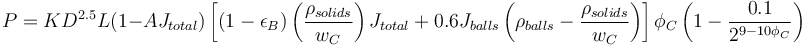# Austin SAG model

## History

The SAG mill model by proposed by Leonard Austin (1990) was largely based on modifications of earlier tumbling mill models by Hogg & Fuerstenau and F. Bond. The model uses a kinetic-potential energy balance to describe the power draw of a mill charge. Many geometric components of the earlier models were fit to empirical relationships measured by Austin.

## Model Form

The power draw model for a SAG mill cylinder of the following form:$P = K D^{2.5} L ( 1 - AJ_{total}) \left [ (1-\epsilon_{B}) \left( \frac{\rho_{solids}}{w_{C}} \right ) J_{total} + 0.6 J_{balls} \left( \rho_{balls} - \frac{\rho_{solids}}{w_C} \right) \right ] \phi_{C} \left( 1 - \frac{0.1}{2^{9-10\phi_C}} \right)$

Where:

• P is the power evolved at the mill shell, kW
• K and A are empirical fitting factors (use 10.6 and 1.03, respectively)
• D is the mill effective diameter (inside the effective liner thickness), m
• L is the mill effective grinding length (also referred to as the 'belly length), m
• Jtotal is the mill total volumetric filling as a fraction (eg. 0.30 for 30%)
• εB is the porosity of the rock and ball load (use 0.3)
• wC is the charge %solids, fraction by weight (use 0.80 Doll, 2013)
• Jballs is the mill volumetric filling of balls as a fraction (eg. 0.10 for 10%)
• ρX is the density of component X, t/m3
• φC is the mill speed as a fraction of critical (eg. 0.75 for 75% of critical)

### Mill cone angles

The model supports flat-ended mills (cone angle of 0°) or a cone angle of 15°. Any other value entered for the cone angle will be treated as 15°.

To account for cone ends of mills, an allowance of 5% is used Doll, 2013 instead of the formula proposed by Austin.

### Percent solids

The model is run with a fixed 80% solids by weight Doll, 2013. This is because the form of the equation proposed by Austin appears to have the %solids term in the wrong place (denominator of the expression rather than the numerator where other models put it). The calibration presented at IMPC 2016 suggests the fixed %solids term is reasonably valid over the range of 60% to 80% solids.

## Model Inputs

the Austin model requests the following mill data:

• mill nominal diameter inside shell
• mill effective grinding length
• ball load as vol percentage (eg. 10)
• ball density
• total charge load as vol percentage (eg. 30)
• pulp percent solids
• mill speed
• liner effective thickness
• cone end angle (only values 0-5 or >5 matter, minor angle changes have no effect)

Motor characteristics are also requested:

• Motor rated power (output shaft)
• Mechanical efficiency of downstream drive (pinions, gearboxes)
• Motor efficiency and any other efficiency factors to the DCS measurement position in the network.
• Motor rated speed, in units of mill RPM (not motor RPM, must multiply by gear ratios).
• Quantity of pinions (and motors)